# Exterior derivative

Let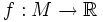be a smooth function on a manifold. The differential (or exterior derivative),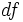, is a covector field on M defined as follows: for v a tangent vector at a point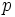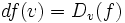i.e.,is the directional derivative of f in the direction v.

Note that ifare a local coordinate system for M at p, then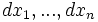define a local co-frame near p. Thus, near p, we may write the differential of f as a linear combination: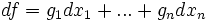In fact, since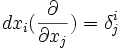, we get that: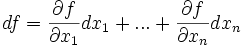## Exterior derivative of differential forms

If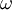is a differential k-form (i.e., a smooth section of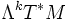), the exterior derivative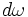is a differential (k+1)-form defined as follows:

If we can writein local coordinates as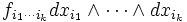then in this coordinate system,equals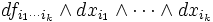More generally, we define the differentialby extending the above definition by linearity.

## Cosmological properties of the differential

The operator d has the important property that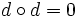. This essentially follows from the equality of mixed partial derivatives. The following simplest example illustrates the general proof: Letbe a smooth function in two variables. Then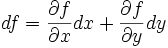Thus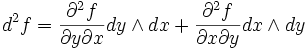Since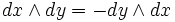, the equality of mixed partials shows that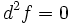.